vn.py量化社区

### 遗传算法原理

1. 随机生成一大推策略参数（称之为族群，族群内的个体对应某一组策略参数）
2. 族群内个体间进行3类活动：个体间两两交叉互换；个体某个参数发生变异；个体繁殖（即直接复制参数）
3. 形成子代
4. 通过目标函数（如最大化夏普比率，最大化总盈亏等）对母代族群和子代族群进行评分
5. 通过特点筛选标准（如NSGA-Ⅱ）从母代和子代中筛选个体，形成第二代族群。（类似进化论中的“自然选择”）
6. 新的族群在特定的评分标准和筛选标准中不断迭代（即重复1-5步骤），得到最优解/次优解。

### 遗传算法代码示例

1.随机生成待优化的策略参数

``````def parameter_generate():
'''
根据设置的起始值，终止值和步进，随机生成待优化的策略参数
'''
parameter_list = []
p1 = random.randrange(4,50,2)      #入场窗口
p2 = random.randrange(4,50,2)      #出场窗口
p3 = random.randrange(4,50,2)      #基于ATR窗口止损窗
p4 = random.randrange(18,40,2)     #基于ATR的动态调仓

parameter_list.append(p1)
parameter_list.append(p2)
parameter_list.append(p3)
parameter_list.append(p4)

return parameter_list``````

1. 设置目标优化函数（收益回撤比和夏普比率）
``````def object_func(strategy_avg):
"""
本函数为优化目标函数，根据随机生成的策略参数，运行回测后自动返回2个结果指标：收益回撤比和夏普比率
"""
# 创建回测引擎对象
engine = BacktestingEngine()
# 设置回测使用的数据
engine.setBacktestingMode(engine.BAR_MODE)      # 设置引擎的回测模式为K线
engine.setStartDate('20170401')                 # 设置回测用的数据起始日期
engine.setEndDate('20181230')                   # 设置回测用的数据起始日期

# 配置回测引擎参数
engine.setSlippage(0.5)
engine.setRate(0.2/100)
engine.setSize(10)
engine.setPriceTick(0.5)
engine.setCapital(1000000)

setting = {'entryWindow': strategy_avg,       #布林带窗口
'exitWindow': strategy_avg,        #布林带通道阈值
'atrWindow': strategy_avg,         #CCI窗口
'artWindowUnit': strategy_avg,}    #ATR窗口

#加载策略
# 运行回测，返回指定的结果指标
engine.runBacktesting()          # 运行回测
#逐日回测
engine.calculateDailyResult()
backresult = engine.calculateDailyStatistics()

returnDrawdownRatio = round(backresult['returnDrawdownRatio'],2)  #收益回撤比
sharpeRatio= round(backresult['sharpeRatio'],2)                   #夏普比率
return returnDrawdownRatio , sharpeRatio``````

3.运行基于Deap库的遗传算法（具体步骤看代码中文注释）

``````#设置优化方向：最大化收益回撤比，最大化夏普比率
creator.create("FitnessMulti", base.Fitness, weights=(1.0, 1.0)) # 1.0 求最大值；-1.0 求最小值
creator.create("Individual", list, fitness=creator.FitnessMulti)

def optimize():
""""""
toolbox = base.Toolbox()  #Toolbox是deap库内置的工具箱，里面包含遗传算法中所用到的各种函数

# 初始化
toolbox.register("individual", tools.initIterate, creator.Individual,parameter_generate) # 注册个体：随机生成的策略参数parameter_generate()
toolbox.register("population", tools.initRepeat, list, toolbox.individual)               #注册种群：个体形成种群
toolbox.register("mate", tools.cxTwoPoint)                                               #注册交叉：两点交叉
toolbox.register("mutate", tools.mutUniformInt,low = 4,up = 40,indpb=0.6)                #注册变异：随机生成一定区间内的整数
toolbox.register("evaluate", object_func)                                                #注册评估：优化目标函数object_func()
toolbox.register("select", tools.selNSGA2)                                               #注册选择:NSGA-II(带精英策略的非支配排序的遗传算法)

#遗传算法参数设置
MU = 40                                  #设置每一代选择的个体数
LAMBDA = 160                             #设置每一代产生的子女数
pop = toolbox.population(400)            #设置族群里面的个体数量
CXPB, MUTPB, NGEN = 0.5, 0.35, 40        #分别为种群内部个体的交叉概率、变异概率、产生种群代数
hof = tools.ParetoFront()                #解的集合：帕累托前沿(非占优最优集)

#解的集合的描述统计信息
#集合内平均值，标准差，最小值，最大值可以体现集合的收敛程度
#收敛程度低可以增加算法的迭代次数
stats = tools.Statistics(lambda ind: ind.fitness.values)
np.set_printoptions(suppress=True)            #对numpy默认输出的科学计数法转换
stats.register("mean", np.mean, axis=0)       #统计目标优化函数结果的平均值
stats.register("std", np.std, axis=0)         #统计目标优化函数结果的标准差
stats.register("min", np.min, axis=0)         #统计目标优化函数结果的最小值
stats.register("max", np.max, axis=0)         #统计目标优化函数结果的最大值

#运行算法
algorithms.eaMuPlusLambda(pop, toolbox, MU, LAMBDA, CXPB, MUTPB, NGEN, stats,
halloffame=hof)     #esMuPlusLambda是一种基于(μ+λ)选择策略的多目标优化分段遗传算法

return pop``````

### 遗传算法效果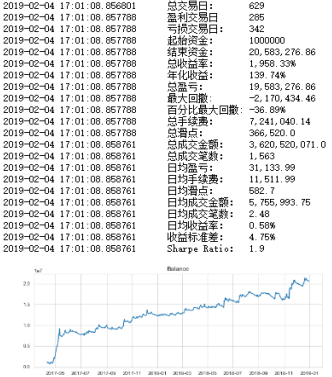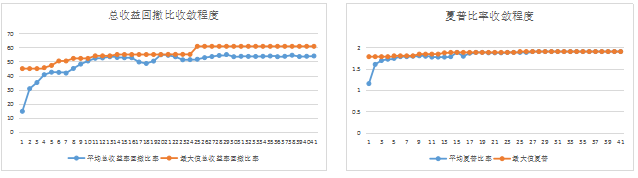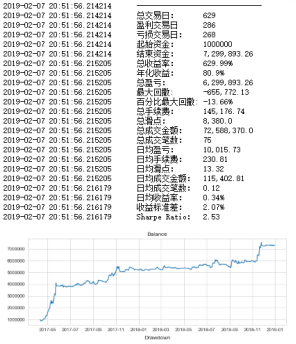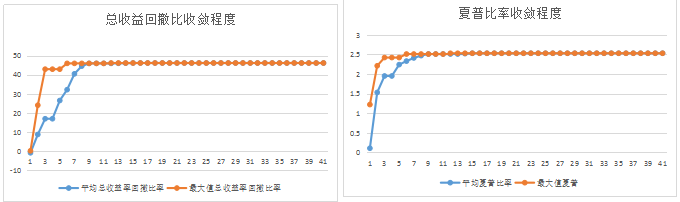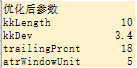### 总结

.....
import multiprocessing
pool = multiprocessing.Pool(processes=multiprocessing.cpu_count())
toolbox.register("map", pool.map)

``````# 运行算法
algorithms.eaMuPlusLambda(pop, toolbox, MU, LAMBDA, CXPB, MUTPB, NGEN, stats,
halloffame=hof)  # esMuPlusLambda是一种基于(μ+λ)选择策略的多目标优化分段遗传算法
....

``````

deap库提供了scoop和multiprocessing库的支持。但是发现在jupter notebook上运行仍然是单进程（纯py文件正常），另外有时会无法输入最终结果pop。

``````def mutArrayGroup(individual,parameterlist, indpb):
size = len(individual)
parameterlist  = parameterlist()
for i in xrange(size):
if random.random() < indpb:
individual[i] = parameterlist[i]

return individual,

toolbox.register("mutate", mutArrayGroup, parameterlist = parameter_generate, indpb=0.6)``````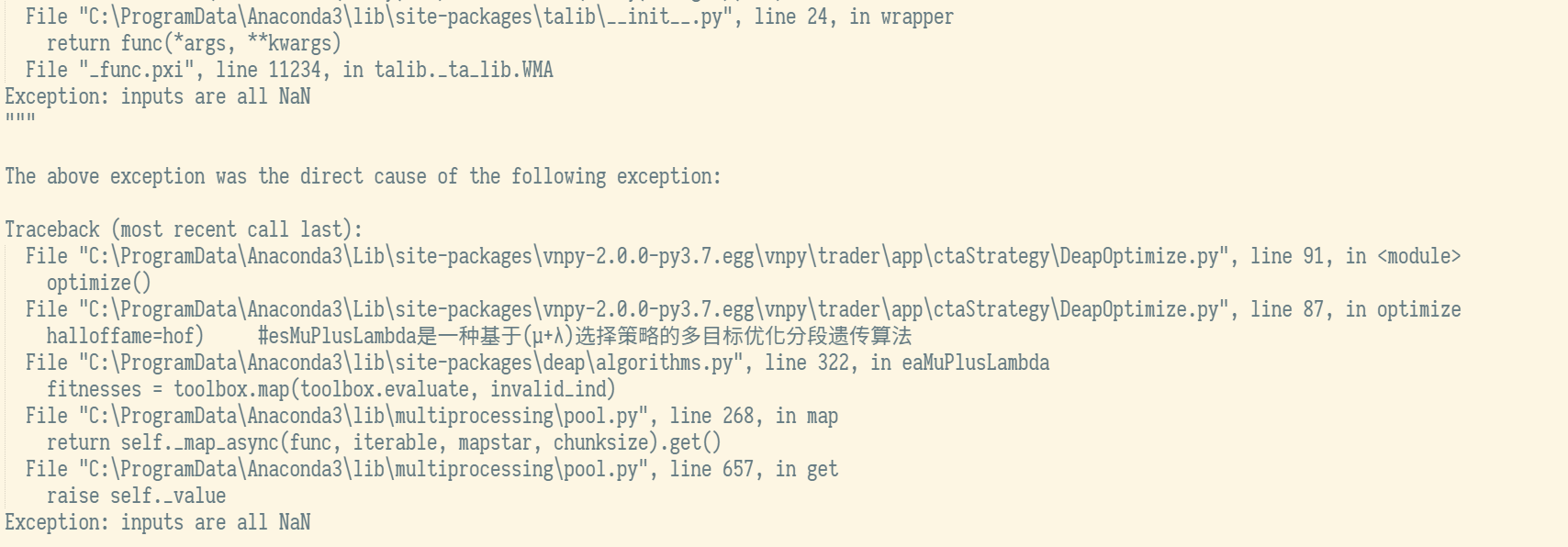``````    try:
profitLossRatio = round(backresult['profitLossRatio'],3)                   #夏普比率                 #夏普比率
sharpeRatio= round(backresult['sharpeRatio'],3)
except:
print("Error ")
sharpeRatio = 0
profitLossRatio = 0                      #收益回撤比

return sharpeRatio,profitLossRatio``````KeKe wrote:

1. Parameterlist 字典； 简化了调用参数和定义对应随机范围问题。只要在Parameterlist 中定义策略参数名称和对应的随机范围就可以，其中两个参数的元祖是两个之间随机数，调用random.uniform()，三个参数元祖是开始结束和中间步进,调用的是random.randrange(), 如果是数组就是在数组中间随机选择。
2. Symbollist 字典，维护回测品种和数据
3. poptoExcel方法，输出一个Excel，包括参数和value；参数可以直接调用。同时把相同项合并。效果如下图

cPickle.PicklingError: Can't pickle <type 'instancemethod'>: attribute lookup builtin.instanceme

[value1,value2,value3...]变成[{key1:value1},{key2,value2},{key2,value2}...]，这样。可以满足交叉，突变要求。进化算法要改下，详情见下文。

@张国平 你加的这个mutArrayGroup会提示mutate(ind) too many values to unpack

@张国平 你加的这个mutArrayGroup会提示mutate(ind) too many values to unpack

@张国平 你加的这个mutArrayGroup会提示mutate(ind) too many values to unpack

[value1,value2,value3...]变成[{key1:value1},{key2,value2},{key3,value3}...]，这样。可以满足交叉，突变要求。进化算法要改下。

``````    def parameter_generate(self):
'''
根据设置的起始值，终止值和步进，随机生成待优化的策略参数
'''
parameter_list = []
for key, value in self.parameterlist.items():
if isinstance(value, tuple):
if len(value) == 3:
parameter_list.append({key:random.randrange(value, value, value)})
elif len(value) == 2:
parameter_list.append({key:random.uniform(value, value)})
elif isinstance(value, list):
parameter_list.append({key:random.choice(value)})
else:
parameter_list.append({key:value})
return parameter_list``````

parameter_list是类似vnpy optimize的格式，不过有所增强。 strategy_avg返回变成了[{key1:value1},{key2,value2},{key3,value3}...]，包含字典的list格式也可以满足交叉和突变方法。

``````    setting = {}
for item in range(len(strategy_avg)):
setting.update(strategy_avg[item])``````

``creator.create("Individual", list, fitness=creator.FitnessMulti, parameterPackage=parameterPackage)``

``````# encoding: UTF-8

"""

"""

from __future__ import division
from __future__ import print_function
from vnpy.trader.app.ctaStrategy.ctaBacktesting import BacktestingEngine, MINUTE_DB_NAME, OptimizationSetting
import random
import numpy as np
from deap import creator, base, tools, algorithms
import multiprocessing
import time, datetime
import pandas as pd

def object_func(strategy_avgTuple):
"""
本函数为优化目标函数，根据随机生成的策略参数，运行回测后自动返回2个结果指标：收益回撤比和夏普比率
"""

strategy_avg = strategy_avgTuple
paraSet = strategy_avgTuple.parameterPackage
symbol = paraSet["symbol"]
strategy = paraSet["strategy"]

# 创建回测引擎对象
engine = BacktestingEngine()
# 设置回测使用的数据
engine.setBacktestingMode(engine.BAR_MODE)  # 设置引擎的回测模式为K线
engine.setStartDate(symbol["StartDate"])  # 设置回测用的数据起始日期
engine.setEndDate(symbol["EndDate"])  # 设置回测用的数据起始日期

# 配置回测引擎参数
engine.setSlippage(symbol["Slippage"])  # 1跳
engine.setRate(symbol["Rate"])  # 佣金大小
engine.setSize(symbol["Size"])  # 合约大小
engine.setPriceTick(symbol["Slippage"])  # 最小价格变动
engine.setCapital(symbol["Capital"])

setting = {}
for item in range(len(strategy_avg)):
setting.update(strategy_avg[item])

engine.clearBacktestingResult()
# 加载策略

engine.initStrategy(strategy, setting)
# 运行回测，返回指定的结果指标
engine.runBacktesting()  # 运行回测
# 逐日回测
# engine.calculateDailyResult()
backresult = engine.calculateBacktestingResult()

try:
capital = round(backresult['capital'], 3)  # 收益回撤比
profitLossRatio = round(backresult['profitLossRatio'], 3)  # 夏普比率                 #夏普比率
sharpeRatio = round(backresult['sharpeRatio'], 3)
except Exception, e:
print("Error: %s, %s" %(str(Exception),str(e)))
sharpeRatio = 0
profitLossRatio = 0  # 收益回撤比
averageWinning = 0  # 夏普比率                 #夏普比率
capital = 0
return capital, sharpeRatio, profitLossRatio

class GeneticOptimizeStrategy(object):
Strategy = BollChannelStrategy
Symbollist ={
"vtSymbol": 'rb0000',
"StartDate": "20140601",
"EndDate": "20141101",
"Slippage": 1,
"Size": 10,
"Rate": 2 / 10000.0,
"Capital": 10000
}
Parameterlist = {
'bollWindow': (10,50,1),       #布林带窗口
'bollDev': (2,10,1),        #布林带通道阈值
'slMultiplier':(3,6),
'barMins':[2,3,5,10,15,20],
}
parameterPackage = {
"symbol":Symbollist,
"strategy":Strategy
}

# ------------------------------------------------------------------------
def __init__(self, Strategy, Symbollist, Parameterlist):
self.strategy = Strategy
self.symbol = Symbollist
self.parameterlist = Parameterlist
self.parameterPackage = {
"strategy":self.strategy,
"symbol":self.symbol
}

creator.create("FitnessMulti", base.Fitness, weights=(1.0, 1.0, 1.0))  # 1.0 求最大值；-1.0 求最小值
creator.create("Individual", list, fitness=creator.FitnessMulti, parameterPackage=parameterPackage)

# ------------------------------------------------------------------------
def parameter_generate(self):
'''
根据设置的起始值，终止值和步进，随机生成待优化的策略参数
'''
parameter_list = []
for key, value in self.parameterlist.items():
if isinstance(value, tuple):
if len(value) == 3:
parameter_list.append({key:random.randrange(value, value, value)})
elif len(value) == 2:
parameter_list.append({key:random.uniform(value, value)})
elif isinstance(value, list):
parameter_list.append({key:random.choice(value)})
else:
parameter_list.append({key:value})
return parameter_list

def mutArrayGroup(self, individual, parameterlist, indpb):
size = len(individual)
paralist = parameterlist()
for i in xrange(size):
if random.random() < indpb:
individual[i] = paralist[i]
return individual,

def optimize(self):
# 设置优化方向：最大化收益回撤比，最大化夏普比率

toolbox = base.Toolbox()  # Toolbox是deap库内置的工具箱，里面包含遗传算法中所用到的各种函数
pool = multiprocessing.Pool(processes=(multiprocessing.cpu_count()-1))
toolbox.register("map", pool.map)

# 初始化
toolbox.register("individual", tools.initIterate, creator.Individual,
self.parameter_generate)  # 注册个体：随机生成的策略参数parameter_generate()
toolbox.register("population", tools.initRepeat, list,
toolbox.individual)  # 注册种群：个体形成种群
toolbox.register("mate", tools.cxTwoPoint)  # 注册交叉：两点交叉
toolbox.register("mutate", self.mutArrayGroup, parameterlist=self.parameter_generate,
indpb=0.6)  # 注册变异：随机生成一定区间内的整数
toolbox.register("evaluate", object_func)  # 注册评估：优化目标函数object_func()
toolbox.register("select", tools.selNSGA2)  # 注册选择:NSGA-II(带精英策略的非支配排序的遗传算法)

# 遗传算法参数设置
MU = 8  # 设置每一代选择的个体数
LAMBDA = 5  # 设置每一代产生的子女数
pop = toolbox.population(20)  # 设置族群里面的个体数量

CXPB, MUTPB, NGEN = 0.5, 0.3, 10 # 分别为种群内部个体的交叉概率、变异概率、产生种群代数
hof = tools.ParetoFront()  # 解的集合：帕累托前沿(非占优最优集)

# 解的集合的描述统计信息
# 集合内平均值，标准差，最小值，最大值可以体现集合的收敛程度
# 收敛程度低可以增加算法的迭代次数
stats = tools.Statistics(lambda ind: ind.fitness.values)
np.set_printoptions(suppress=True)  # 对numpy默认输出的科学计数法转换
stats.register("mean", np.mean, axis=0)  # 统计目标优化函数结果的平均值
stats.register("std", np.std, axis=0)  # 统计目标优化函数结果的标准差
stats.register("min", np.min, axis=0)  # 统计目标优化函数结果的最小值
stats.register("max", np.max, axis=0)  # 统计目标优化函数结果的最大值
# 运行算法
algorithms.eaMuPlusLambda(pop, toolbox, MU, LAMBDA, CXPB, MUTPB, NGEN, stats,
halloffame=hof, verbose=True)  # esMuPlusLambda是一种基于(μ+λ)选择策略的多目标优化分段遗传算法

return pop

def poptoExcel(self, pop, number = 1000, path = "C:/data/"):
#按照输入统计数据队列和路径，输出excel，这里不提供新增模式，如果想，可以改
path = path +  self.strategy.className + "_" + self.symbol[ "vtSymbol"] + str(datetime.date.today())+ ".xls"
summayKey = ["StrategyParameter","TestValues"]
best_ind = tools.selBest(pop, number)
dft = pd.DataFrame(columns=summayKey)

for i in range(0,len(best_ind)-1):
if i == 0:
# new = pd.DataFrame([{"StrategyParameter":self.complieString(best_ind[i])},{"TestValues":best_ind[i].fitness.values}], index=["0"])
dft = dft.append([{"StrategyParameter":self.complieString(best_ind[i]),"TestValues":best_ind[i].fitness.values}], ignore_index=True)
elif str(best_ind[i-1]) == (str(best_ind[i])):
pass
else:
#new = pd.DataFrame({"StrategyParameter":self.complieString(best_ind[i]),"TestValues":best_ind[i].fitness.values}, index=["0"])
dft = dft.append([{"StrategyParameter":self.complieString(best_ind[i]),"TestValues":best_ind[i].fitness.values}], ignore_index=True)

print("回测统计结果输出到" + path)

def complieString(self,individual):
setting = {}
for item in range(len(individual)):
setting.update(individual[item])
return str(setting)

if __name__ == "__main__":
Strategy = BollChannelStrategy

Symbollist ={
"vtSymbol": 'rb0000',
"StartDate": "20140601",
"EndDate": "20141101",
"Slippage": 1,
"Size": 10,
"Rate": 2 / 10000.0,
"Capital": 10000
}
Parameterlist = {
'bollWindow': (10,50,1),       #布林带窗口
'bollDev': (2,10,1),        #布林带通道阈值
'slMultiplier':(3,6),
'barMins':[2,3,5,10,15,20],
}
parameterPackage = {
"symbol":Symbollist,
"parameterlist":Parameterlist,
"strategy":Strategy
}

GE = GeneticOptimizeStrategy(Strategy,Symbollist,Parameterlist)
GE.poptoExcel(GE.optimize())

print("-- End of (successful) evolution --")``````